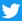## Kinematic Data:

initial
position (ft)
initial
velocity (ft/s)
average
acceleration (ft/s2)
xo = -1.546 vxo = 6.983 ax = -10.652
yo = 50.00 vyo = -130.096 ay = 30.456
zo = 6.4 vzo = -3.843 az = -22.578

Initial Speed (mph): 88.9
Speed at the front of home plate (mph): 81.1
Position at the front of home plate (ft):
x = 0.372, y = 1.417, and z = 3.167

Maintained by Dr. Baseball, Ph.D. ()